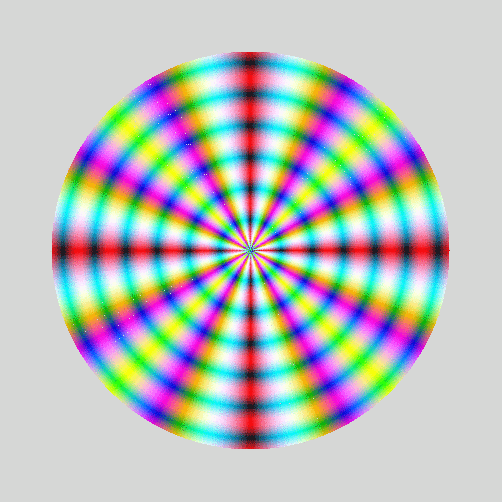The X and Y values for a circle can be calculated with the formulae

``````
``````

The value of `radians` varies between 0 and 2*pi.

The image below is constructed by calculating circles with radii from 1 to 200 pixels.

The pixel color is set with these formulas:

``````